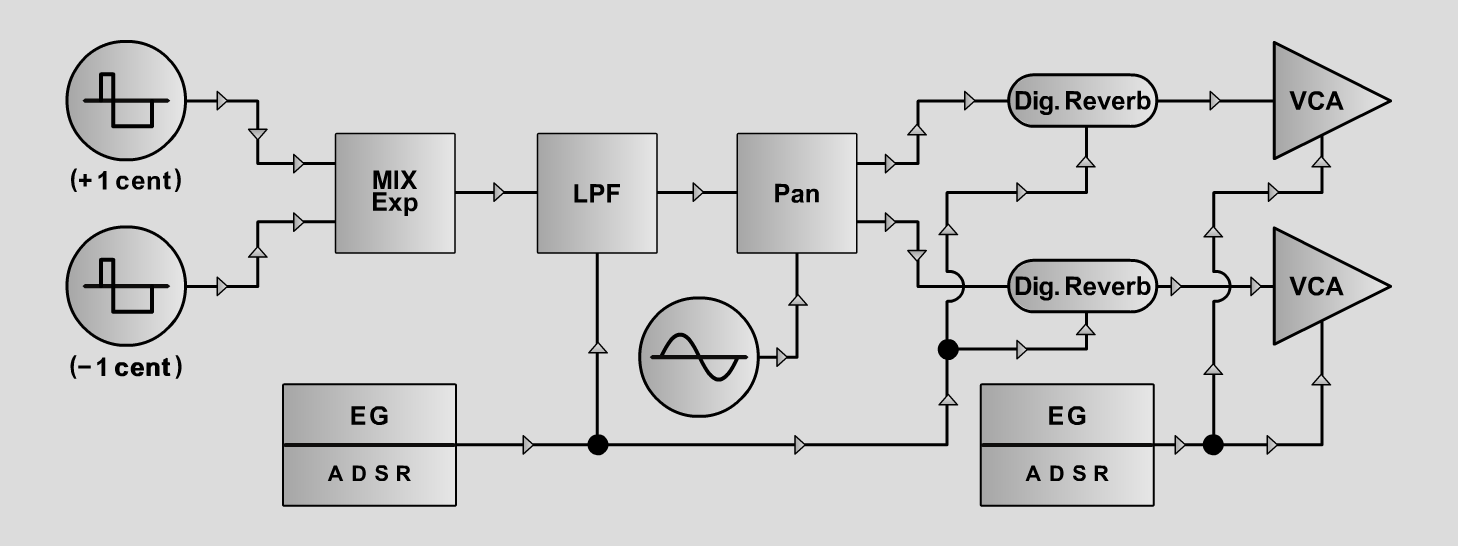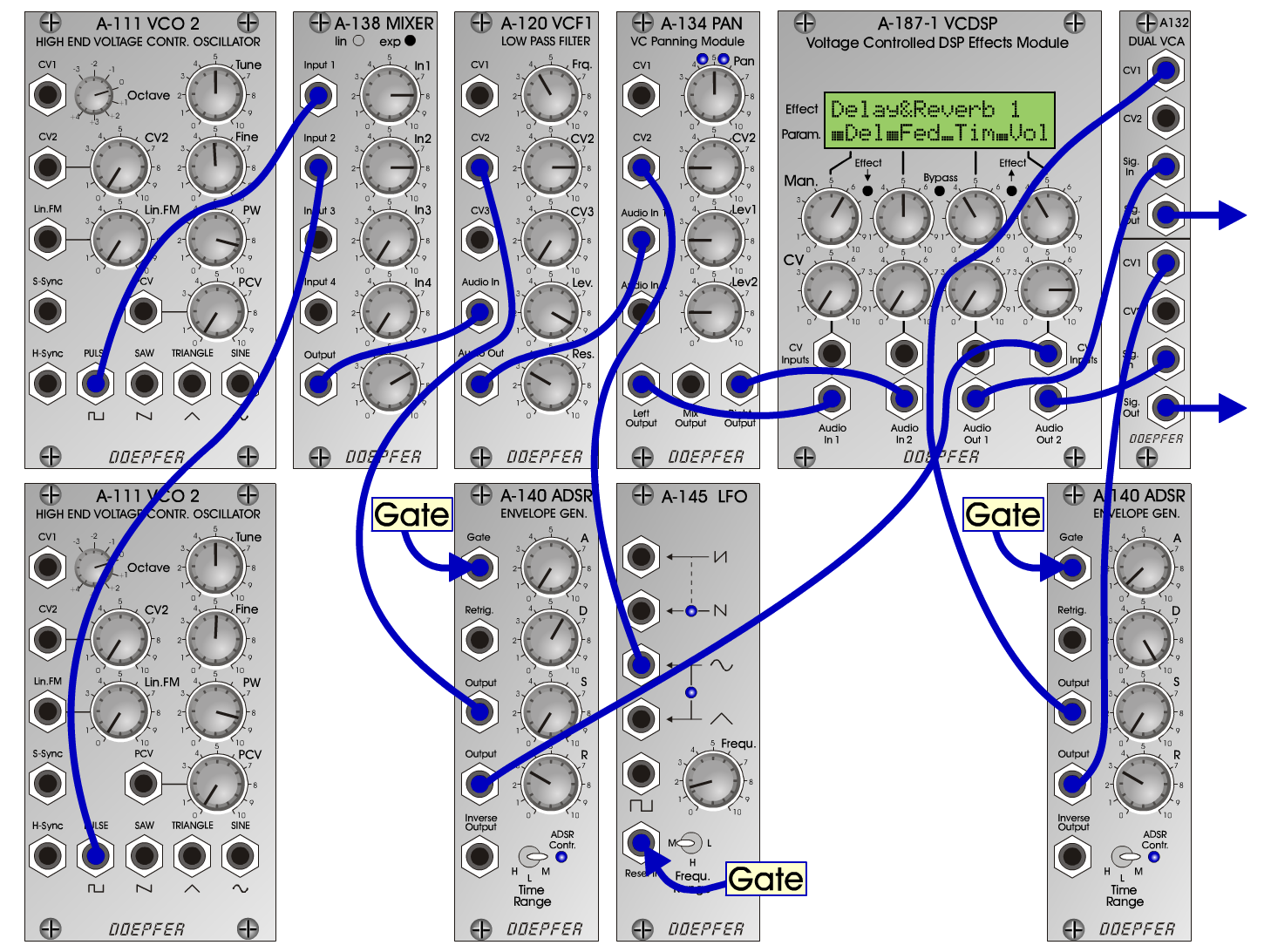RevalgoS y n t hD o e p f e r A - 100 Connections: Settings: Revalgo 1 A-111/1 (Pulse) <=> A-138exp (Input 1)  A-111/2 (Pulse) <=> A-138exp (Input 2)  A-138exp (Output) <=> A-120 (Audio In)  A-120 (Audio Out) <=> A-134 (Audio In 1)  A-134 (Left Out) <=> A-187-1 (Audio In 1)  A-134 (Right Out) <=> A-187-1 (Audio In 2)  A-187-1 (Audio Out 1) <=> A-132up (Sig In)  A-187-1 (Audio Out 2) <=> A-132down (Sig In)  A-132up (Sig Out) <=> Amp left  A-132down (Sig Out) <=> Amp right  A-140/1 (Output 1) <=> A-132up (CV 1)  A-140/1 (Output 2) <=> A-132down (CV 1)  A-140/2 (Output 1) <=> A-120 (CV2)  A-140/2 (Output 2) <=> A-187-1 (CV In 4)  A-145 (Sine) <=> A-134 (CV 2)  Gate <=> A-145 (Reset In) A-138exp (In 1 = 8, In 2 = 8, Out = 7)  A-120 (Freq = 4, CV2 = 8, Lev = 9, Res = 3)  A-134 (Pan = 5, CV 2 = 2, Lev 1 = 2, Lev 2 = 2)  A-140/1 (A = 0.5, D = 10, S = 0, R = 3, Range = M)  A-140/2 (A = 0, D = 6, S = 0, R = 3, Range = M)  A-145 (Frq = 1.5, Range = M)  A-187-1 (Effect = Delay & Reverb 1;  Man1 = 6, Man2 = 5, Man3 = 4, Man4 = 4,  CV1 = 0, CV2 = 0, CV3 = 0, CV4 = 8) Revalgo 2 A-138exp (In 1 = 8, In 2 = 8, Out = 7)  A-120 (Freq = 4, CV2 = 8, Lev = 9, Res = 3)  A-134 (Pan = 5, CV 2 = 2, Lev 1 = 2, Lev 2 = 2)  A-140/1 (A = 0.5, D = 10, S = 0, R = 3, Range = M)  A-140/2 (A = 0, D = 6, S = 0, R = 3, Range = M)  A-145 (Frq = 1.5, Range = M)  A-187-1 (Effect = Chorus/Flanger/Echo 2;  Man1 = 4, Man2 = 5, Man3 = 7, Man4 = 5,  CV1 = 0, CV2 = 0, CV3 = 0, CV4 = 10) Revalgo 0 A-138exp (In 1 = 8, In 2 = 8, Out = 7)  A-120 (Freq = 4, CV2 = 8, Lev = 9, Res = 3)  A-134 (Pan = 5, CV 2 = 2, Lev 1 = 2, Lev 2 = 2)  A-140/1 (A = 0.5, D = 10, S = 0, R = 3, Range = M)  A-140/2 (A = 0, D = 6, S = 0, R = 3, Range = M)  A-145 (Frq = 1.5, Range = M)  A-187-1 (Bypass = On, without effect) Tuning: A-111/1 => + 1 cent; A-111/2 => - 1 cent Josef MuellerSound samples Bach Cello-Suite 1, Prelude (BWV 1007) Revalgo 0 Revalgo 1 Revalgo 2 Revalgo 0 (with reverb) Revalgo 1 (with reverb) Revalgo 2 (with reverb) Revalgo 0 A Revalgo 0 B Revalgo 0 C Revalgo 1 A Revalgo 1 B Revalgo 1 C Revalgo 2 A Revalgo 2 B Revalgo 2 C